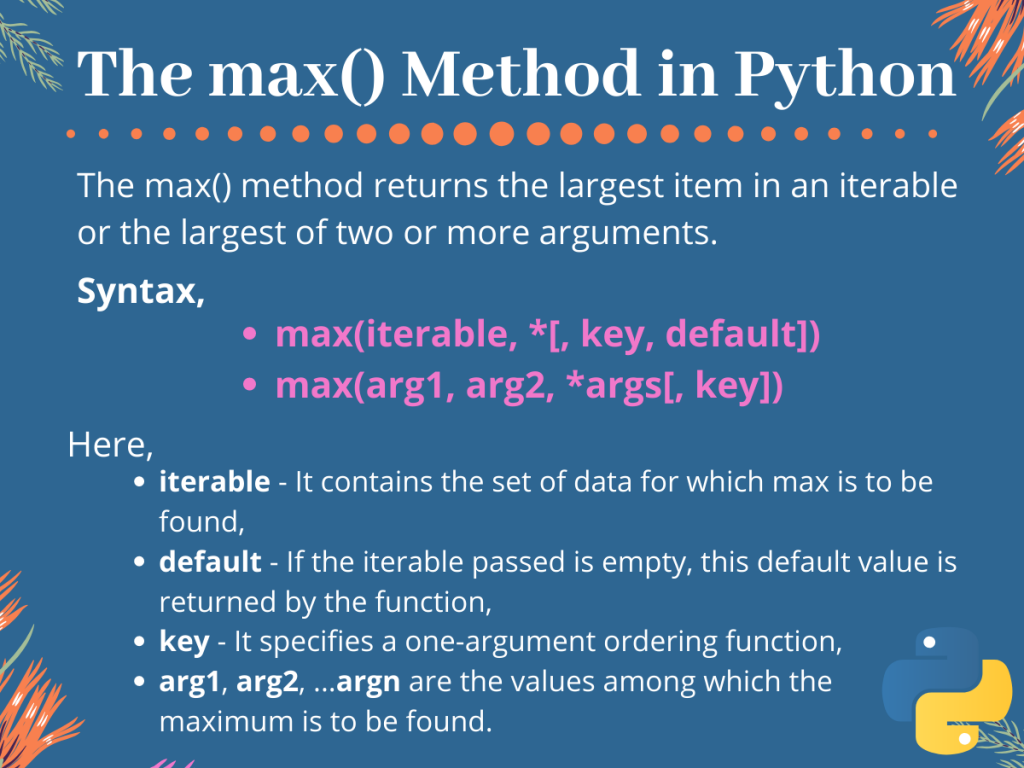# The Python max() Method## Introduction

In this tutorial, we are going to understand the use of the Python max() method. Basically, the Python `max()` method returns the maximum value among the set of passed values or the elements of the passed iterable.

## Using the Python max() Method

Below is the syntax for using the Python `max()` method to find the largest value in an iterable,

```max(iterable, *[, key, default])
```

Here,

• iterable is the object that contains the values for which the largest is to be found,
• key specifies the one-argument ordering function,
• And the default is the default value returned by the method if the passed iterable is empty.

For finding largest among two or more values passed as arguments,

```max(arg1, arg2, *args[, key])
```

Here,

• arg1, arg2, …. argn are n values among which, the `max()` method would return the largest value,
• And key again is the ordering function.

## Working with the Python max() Method

We can use the `max()` method in various ways to find the maximum or largest of a given iterable or for two or more arguments.

Let us see how the method works with an iterable object, two or more values, with specified key function and for multiple iterable objects passed as arguments.

## With iterable object

For the example below, we consider a list with some values in it for which we are to find the largest element. Look at the code given below carefully.

```#initialisation of list
list1 = [ 1,3,4,7,0,4,8,2 ]

#finding max element
print("max value is : ", max(list1,default=0))
```

Output:

```max value is :  8
```

As we can see, for the above code we initialize a list, `list1` and directly pass it to the `max()` method with the default value set to 0. The function returns 8 as it is the largest value.

If the list was empty, the function would have passed the default value, which is 0.

## Passing Two or More Values to the Python max() Method

When two or more values are passed to the `max()` method, it returns the maximum or largest of them all. These arguments can be integers, floating-point values, characters or even strings.

Let us take an example,

```print("max value is : ", max(6,1,73,6,38))
```

Output:

```max value is :  73
```

As desired, we get the maximum value, 73.

## With key function

As we mentioned earlier, the key is a one-lined ordering function on the basis of which the maximum value among a set of values is to be found.

For example, if we want to find a tuple from a list of tuples, which has the largest value of the 2nd element. Let us see how we can do that.

```#initialisation of variables
list1 = [(9,2,7), (6,8,4), (3,5,1)]

def f(tuple_1):
return tuple_1

print("max : ", max(list1, key=f))
```

Output:

```max :  (6, 8, 4)
```

Here, `f()` is a user-defined function that returns the 2nd element of the passed tuple. Passing this function as a key to the `max()` method ensures that a tuple is returned with the largest 2nd element. For our example, it is ( 6, 8, 4).

## Passing Multiple Iterables as Arguments

As we stated earlier, the Python max() method can also return the largest of multiple iterable elements as arguments. These arguments can be iterable like string, character, tuple, list or etc..

By default, the `max()` method returns the object with the maximum 0th element for lists, tuples, etc. And for strings, it compares the first character of each string passed.

Below we have taken an example for tuples. Look at the code carefully.

```#initialisation of variables
tuple1 = (5,23,7)
tuple2 = (4,1,7)
tuple3 = (7,37,1)

print("max : ", max(tuple1,tuple2,tuple3))
```

Output:

```max :  (7, 37, 1)
```

For this example, three tuples with some initial values have been directly passed to the `max()` method. Which returns the tuple with the largest first element i.e., (7, 37, 1).

## Conclusion

So in this tutorial, we learned about the Python `max()` method, its uses as well as its working.

Please remember, that if the default value is not set and an empty iterable is passed as arguments to the `max()` function raises a ValueError.

For any further questions related to this topic feel free to use the comments below.